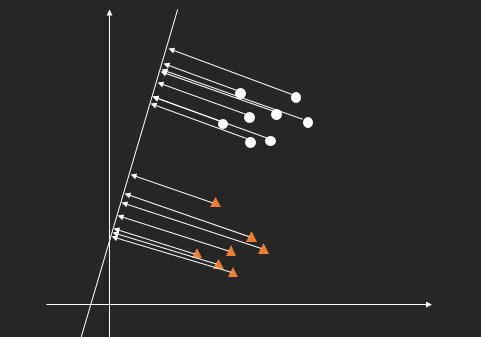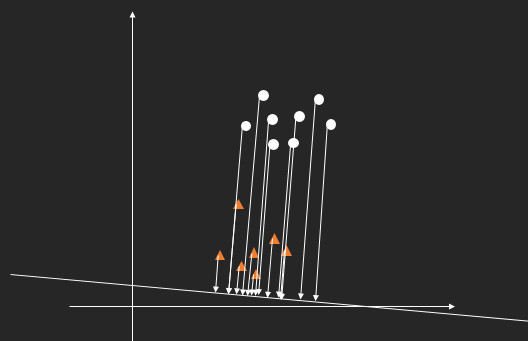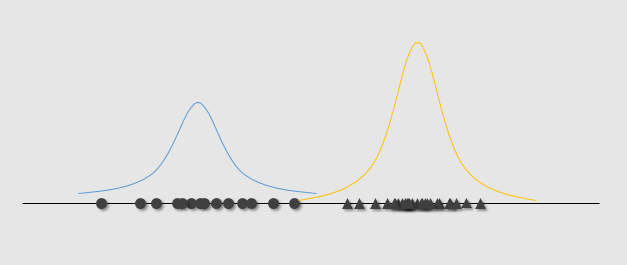Fenrier Lab

# Fisher 线性判别分析$\mathbf{w}^T \mathbf{x} + b = 0$

$\mathbf{x} =\mathbf{x}_p + r\frac{\mathbf{w}}{||\mathbf{w}||}$

$y(\mathbf{x}) = \mathbf{w}^T \mathbf{x} +b$

\begin{aligned} y(\mathbf{x}) &= \mathbf{w}^T (\mathbf{x}_p +r\frac {\mathbf{w}}{||\mathbf{w}||}) + b\\ &= \mathbf{w}^T \mathbf{x}_p + b + r ||\mathbf{w}||\\ &=r ||\mathbf{w}|| \end{aligned}

$y(\mathbf{x}) = r$

$S_i^2 = \sum_{\mathbf{x}\in A_i} (y(\mathbf{x}) - \bar{r}_i)^2,\quad i = 1,2$

$S^2 = S_1^2 + S_2^2$

$(\bar{r}_1 - \bar{r}_2)^2$

$J(\mathbf{w}) = \frac{(\bar{r}_1 - \bar{r}_2)^2}{ S_1^2 + S_2^2}$

\begin{aligned} \bar{r}_1 - \bar{r}_2 &= \frac 1 {n_1} \sum_{\mathbf{x}\in A_1} y(\mathbf{x}) - \frac 1 {n_2} \sum_{\mathbf{x}\in A_2} y(\mathbf{x})\\ &= \frac 1 {n_1} \sum_{\mathbf{x}\in A_1}(\mathbf{w}^T \mathbf{x} + b) - \frac 1 {n_2} \sum_{\mathbf{x}\in A_2}(\mathbf{w}^T \mathbf{x} + b)\\ &=\mathbf{w}^T \left( \frac 1 {n_1} \sum_{\mathbf{x}\in A_1}\mathbf{x} - \frac 1 {n_2} \sum_{\mathbf{x}\in A_2} \mathbf{x}\right) \end{aligned}\\

$m_1 = \frac 1{n_1} \sum_{\mathbf{x} \in A_1} \mathbf{x}\\ m_2 = \frac 1{n_2} \sum_{\mathbf{x} \in A_2} \mathbf{x}$

\begin{aligned} (\bar{r}_1 - \bar{r}_2)^2 &= \mathbf{w}^T (m_1 - m_2) (m_1^T - m_2^T)\mathbf{w}\\ &=\mathbf{w}^T S_b \mathbf{w} \end{aligned}

\begin{aligned} S_1^2 &= \sum_{\mathbf{x} \in A_1} (y(\mathbf{x} ) - \bar{r}_1)^2\\ &= \sum_{\mathbf{x} \in A_1} (\mathbf{w}^T \mathbf{x} + b - \frac 1 {n_1}\sum_{\mathbf{x} \in A_1} (\mathbf{w}^T \mathbf{x} + b))^2\\ &= \sum_{\mathbf{x}\in A_1}(\mathbf{w}^T \mathbf{x} - \frac 1 {n_1} \mathbf{w}^T\sum_{\mathbf{x}\in A_1} \mathbf{x})^2\\ &= \mathbf{w}^T \sum_{\mathbf{x}\in A_1}(\mathbf{x} - \frac 1 {n_1} \sum_{\mathbf{x}\in A_1}\mathbf{x})(\mathbf{x}^T - \frac 1 {n_1} \sum_{\mathbf{x} \in A_1}\mathbf{x}^T) \mathbf{w}\\ &=\mathbf{w}^T \sum_{\mathbf{x} \in A_1} (\mathbf{x} - m_1)(\mathbf{x}^T - m_1^T)\mathbf{w} \end{aligned} \begin{aligned} S_1^2 +S_2^2 &= \mathbf{w}^T \left( \sum_{\mathbf{x} \in A_1} (\mathbf{x} - m_1)(\mathbf{x}^T - m_1^T) +\sum_{\mathbf{x} \in A_2} (\mathbf{x} - m_2)(\mathbf{x}^T - m_2^T)) \right)\mathbf{w}\\ &= \mathbf{w}^T S_w \mathbf{w} \end{aligned}

$J(\mathbf{w}) = \frac{\mathbf{w}^T S_b \mathbf{w}} {\mathbf{w}^T S_w \mathbf{w}}$

$L(\mathbf{w}, \lambda) = \mathbf{w}^T S_b \mathbf{w} - \lambda(\mathbf{w}^T S_w \mathbf{w} - 1)$

$\nabla_{\mathbf{w}} L = S_b \mathbf{w} - \lambda S_w \mathbf{w} = 0$

$S_w^{-1} S_b \mathbf{w}^* = \lambda \mathbf{w}^*$

$S_b = (m_1 - m_2) (m_1 - m_2)^T$

$\mathbf{w}^* = S_w^{-1} (m_1 - m_2) \frac{(m_1 -m_2)^T \mathbf{w}^* }{\lambda}$

$\mathbf{w}^* = S_w^{-1} (m_1 - m_2)$

$S_w \mathbf{w}^* = m_1 - m_2$

$\mathbf{w}^* = \frac{\mathbf{w}^* } {||\mathbf{w}^* ||}$

$\mathbf{w}^{* {T}} \mathbf x +b = 0$

$\{d_i = \mathbf{w}^{* T} \mathbf{x}_i | \mathbf{x}_i \in A\}$

$\{(\mathbf{x}_i ,\, y) |\, i \in \{1,2,..,n\},\,\, y \in \{0,1\}\}$

$\{(d_i ,\, y) |\, i \in \{1,2,..,n\},\,\, y \in \{0,1\}\}$

$p(x) = \frac{1}{\sqrt{2 \pi}\sigma} \exp\left(- \frac{(x-\mu)^2}{2\sigma^2}\right)$

\begin{aligned} &\mu = \frac 1 n \sum x_i\\ &\sigma^2 = \frac{1}{n} \sum (x_i - \mu)^2 \end{aligned}

\begin{aligned} &\mu_i = \frac 1 {n_i} \sum_{\mathbf{x} \in A_i} d\\ &\sigma_i^2 = \frac{1}{n_i} \sum_{\mathbf{x} \in A_i} (d - \mu)^2 \end{aligned}$p(x|y=0)\sim N(\mu_1, \sigma_1)\\ p(x|y=1)\sim N(\mu_2, \sigma_2)$